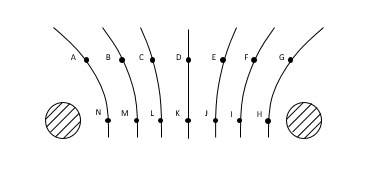# Problem: Two spherical charged conductors placed 6 cm apart produce the equipotential surfaces shown in the figure. The change in potential between each surface is constant, with the 0V line being equidistant from the two charges, and some +V0 at the left-most surface.What is the best comparison of the electric fields at points A and F?A. Both point to the leftB. Both point to the rightC. Field at A points to the right and field at F points to the leftD. Field at A points to the left and field at F points to the rightE. Cannot know without knowing nature of the charges

###### FREE Expert SolutionView Complete Written Solution
###### Problem Details

Two spherical charged conductors placed 6 cm apart produce the equipotential surfaces shown in the figure. The change in potential between each surface is constant, with the 0V line being equidistant from the two charges, and some +V0 at the left-most surface.

What is the best comparison of the electric fields at points A and F?

A. Both point to the left

B. Both point to the right

C. Field at A points to the right and field at F points to the left

D. Field at A points to the left and field at F points to the right

E. Cannot know without knowing nature of the charges